10. WAVE OPTICS

Q.1. Two electric lines of force cannot intersect each other. Why ? Sketch the lines of force for two equal negative charges q1 and q2 placed at points A and B, separated by a small distance in air.

Ans ⇒ Let two electric lines of force I and II intersect point say PA and PB. Two different tangents at a point will mean two different direction of electric field at the same point which is not possible. So, two electric lines of force cannot intersect.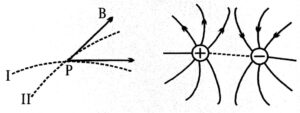The sketch of lines of force of two equal negative charges q1 and q2 placed at A and B separated by a small distance in air is shown in the figure.

Q.2. In a double slit interference experiment, the two coherent beams have slightly different intensities I and I + δI (δI << I). Show that the resultant intensity at the maxima is nearly 4I while that at the minima is nearly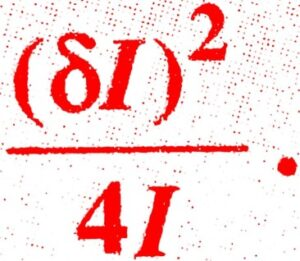Ans ⇒ The intensity due to constructive and distructive superposition are respectively,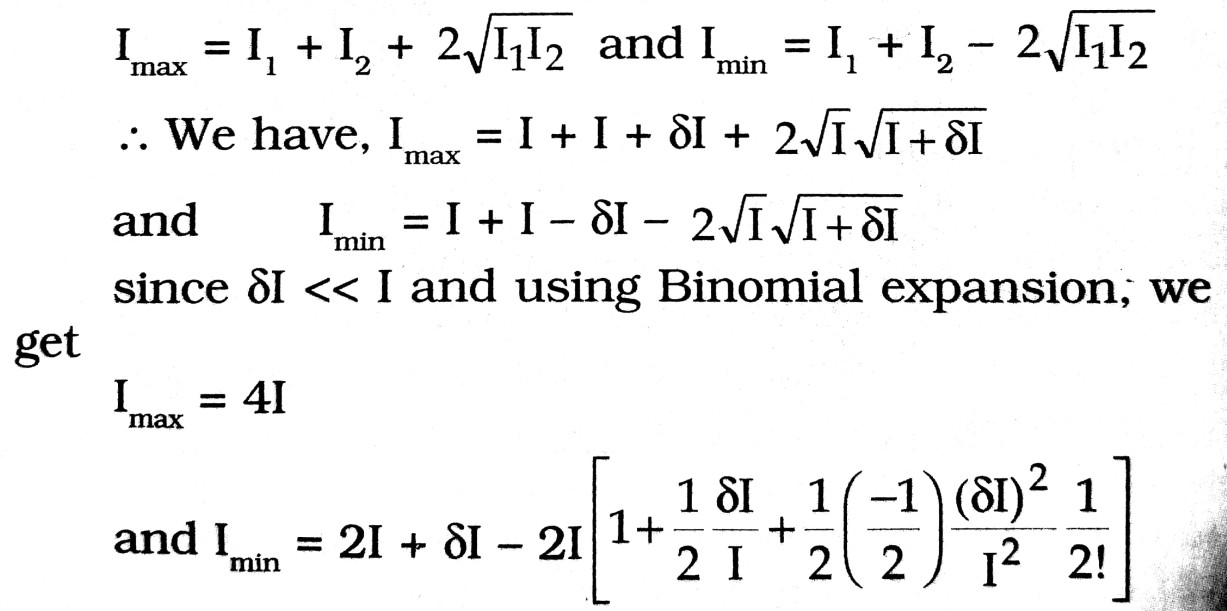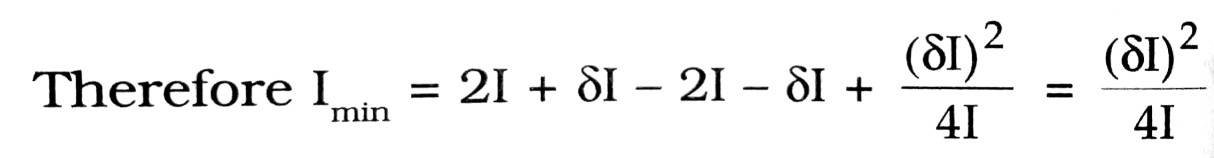Q.3. What are coherent sources of light ? When two slits in Young’s double slit experiment are illuminated by two different sodium lamps emitting light of the same wavelength. Then it is no interference pattern observed why ?

Ans ⇒ Sources of light which emit waves of zero or constant phase difference between them are called coherent sources. Coherence can not be obtained from two independent sources because their phase difference does not remain constant.

Q.4. How can spherical aberration be minimized ?

Ans ⇒ The magnitude of spherical aberration for a lens depends on the radii of curvature and the object distance. It can be minimized by the following methods

(a) By using a diaphragm in front of the lens to reduce its effective aperture. It allows only a narrow pencil of rays to pass through the lens. However, a sharper image is obtained at the cost of the brightness of the image.

(b) Spherical aberration can be reduced by proper choice of curvatures of the lens surface.

(c) Spherical aberration is less if the total deviation of the rays in distribu- ted over the two surfaces of the lens. In case of a plano-convex lens, when the curved surface faces the incident rays, the aberration is much smaller than the case when the plane surface faces the incident rays.

Q.5. Explain the essential difference between interference and diffraction.

Ans ⇒ According to Huygens principle each point on a wave-front is a source of secondary wavelets. Since the wave-front at an instant is the locus of all the immediately neighbouring particles which are in the same phase of vibration at that instant, the secondary sources on the wave front might be termed as coherent sources. The interference of the secondary wavelets derived from a particular wave-front, produces a phenomenon known as diffraction. Thus interference effect is due to superposition of the secondary waves coming from the different parts of the same wave-front.

Q.6. Show that the range of transmission ‘d’ of a T.V. tower of length ‘h’ is given by the relation d = √rh , where r is the radius of the earth.

Ans ⇒ The figure shows a T.V. transmitting antenna of height AB = h located at A on the surface of the earth of radius R. The signal transmitted can be received within a circle of radius AS on the surface of the earth. Now in the right angled triangle OBS.
OB2 = OS2 + BS2
Now BS = AS = d. Then
(R + h)2 = d2 + R2
or, d2 = h + hR
Since h << R, neglecting h2,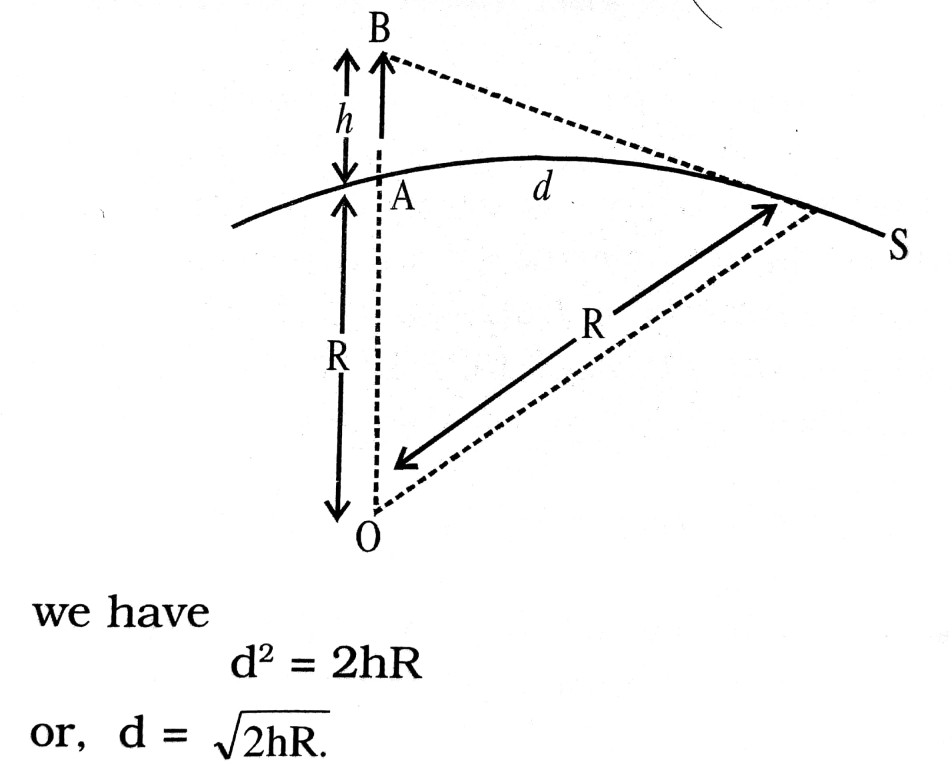Q.7. What is diffraction of light ? Draw a graph showing the variation of intensity with angle in a single slit diffraction experiment. Write one feature which distinguishes the observed pattern from the double slit interference pattern.
How would the diffraction pattern of a single slit be affected when :
(i) The width of the slit is decreased ?
(ii) The monochromatic source of light is replaced by a source of white light ?

Ans ⇒ Diffraction of light : Phenomenon of bending of light round the corners of an obstacle or aperture is called diffraction. Fig. showing the variation of intensity with angle in single slit diffraction experiment.In diffraction pattern, the brightness of successive bright fringes from the centre goes on decreasing whereas in interference pattern all bright fringes are equally bright and have the same width.

Width of central maxima = 2λD/d
(i) When the width of slit (d) is decreased, angular width increases.
(ii) When the monochromatic source of light is replaced by source of white light, the diffraction pattern is coloured. The central maxima is bright but other bands are coloured. Since band width αλ.
∴             βred  >  βviolet

Q.8. What is interference of light ? Write two essential conditions for sustained interference pattern to be produced on the screen.
Draw a graph showing the variation of intensity versus the position on the screen in Young’s experiment. When (a) both the slits are opened and (b) one of the slits is closed.
What is the effect on the interference pattern in Young’s double slit experiment when :
(i) Screen is moved closer to the plane of slits ?
(i) Separation between two slits is increased. Explain your answer in each case.

Ans ⇒ Interference of light : Phenomenon of redistribution of light energy in a medium on account of superposition of light waves from two coherent sources is called interference. Essential condition for interference pattern :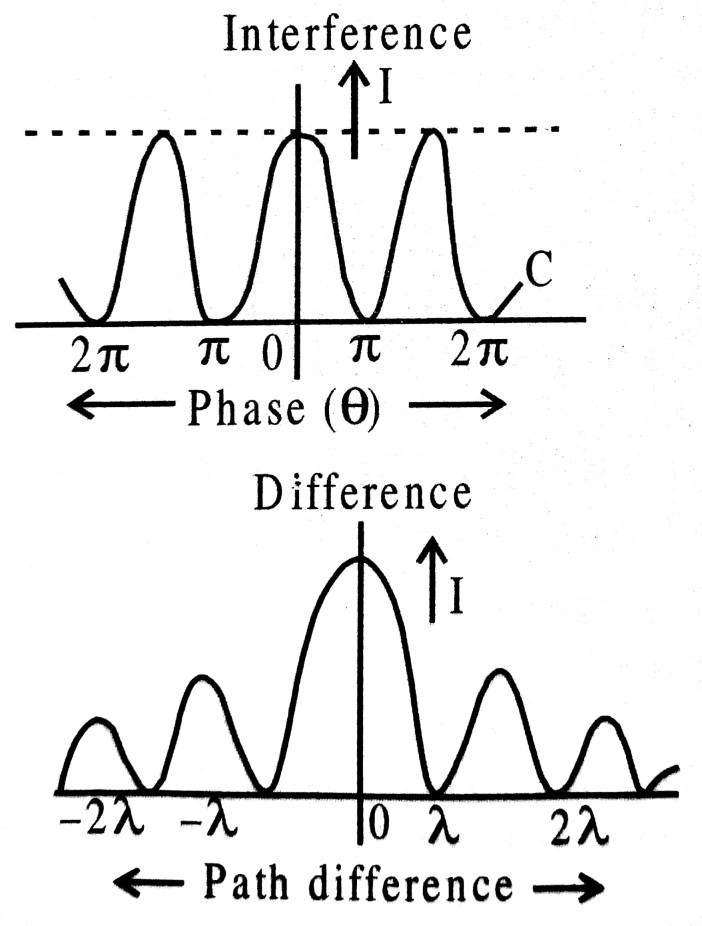(i) The two sources should be produce wave of same wavelength.
(ii) The two sources should be coherent.
(iii) Amplitude of two wave should be either equal or nearly equal.
The Figures shows the variation of intensity verses position Young’s experiment.
(a) When both the slits are opened.
(b) If we close one of the slits, diffraction pattern is obtained
Fringe width is given by
β = λD/d, Therefore
(a) When screen is moved closer to the plane of the slits, width decreases (β α D).
(b) When separation between two slits, increases, the fringe width will decrease.

Q.9. State Huygen’s postulates of wave theory. Sketch the wave-front emerging from a
(i) point source of light and (ii) linear source of light like a slit.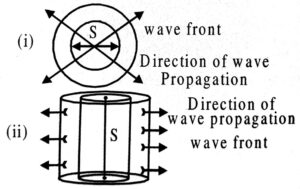Ans ⇒ Postulates : Every point on primary wave front acts a source of secondary wavelets, the forward envelope of which given new wave-front.

Q.10. What are coherent sources ? How does the width of interference fringes in Young’s slit experiment change when
(a) the distance between the slits and screen is decreased ?
(b) frequency of the source is increased ? Justify your answer in each case,

Ans ⇒ Two sources which produce waves of some frequency with zero or constant phase difference between them are called coherent sources.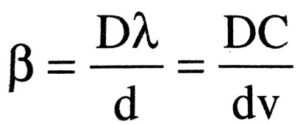(a) When the distance D between the slits and the screen is increased, the fringe width ß decreases, because β α D.
(b) When the frequency v of the source is increased, the fringe with β decreases because β α 1/v.

Q.11. In deriving the single slit diffraction pattern, it was stated that the intensity is zero at angles of nλ/a. Justify this by suitable dividing the slit to bring out the cancellation.

Ans ⇒ Let us suppose that we have n slits each of width a so that a’ = a/n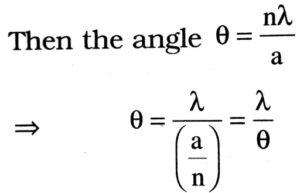Therefore, each of the n slits of width a each sends zero intensity in the direction θ. As a result, the net resultant of intensity due to n such slits is zero.

Q.12. What is main difference between Huygen’s eyepiece & Ramsden eye pieces.

Ans ⇒ Huygen’s eye piece form little curved image. It is used in zoological & botanical observations. It provides poor relief to eye. It gives condition of minimum spherical aberration.
The image formed by Ramsden eye piece is plane & provides more relief. It is implied is spectrometer, compound microscope & telescope. In This case micrometer is set near cross wire.

Q.13. If the incident ray in Young’s experiment be white. What is the colour of central Fringe ? What is the colour of the fringe nearest to the central fringe ?

Ans ⇒ The colour of central fringe is white because all the waves constructively interfere at this point.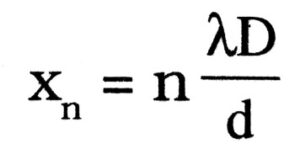Shows that violet colour will be nearest to central fringe.

Q.14. What do you mean by polarization of light?

Ans ⇒ When general light comes out from Tourmaline crystal, its nature is not same as it was before enterance. There is change in intensity. The light emitted is not symmetrical about direction of propagation. There vibrations are confined in a plane normal to the direction of propagation. This light is called polarised light and phenomena is called polarisation.

Q.15. Why red signals are used as danger sign ?

Ans ⇒ According to Rayleigh’s scatting formula the entering of scattered light is proportional to the fourth power of wavelength λ of light.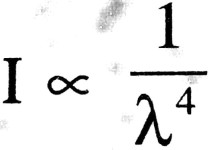In the spectrum of visible light wavelength of red component is maximum, hence due to less scattering red right is observed from very long distance.

## Class 12th physics Subjective question in English

 S.N Physics Short Type Question English Medium 1. ELECTRIC CHARGES AND FIELDS 2. LECTROSTATIC POTENTIAL AND CAPACITANCE 3. CURRENT ELECTRICITY 4. MOVING CHARGES AND MAGNETISM 5. MAGNETISM AND MATTER 6. ELECTROMAGNETIC INDUCTION 7. ALTERNATING CURRENT 8. ELECTROMAGNETIC WAVES 9. RAY OPTICS AND OPTICAL INSTRUMENTS 10. WAVE OPTICS 11. DUAL NATURE OF MATTER AND RADIATION 12. ATOMS 13. NUCLEI 14. SEMI CONDUCTOR ELECTRONICS 15. COMMUNICATION SYSTEMS